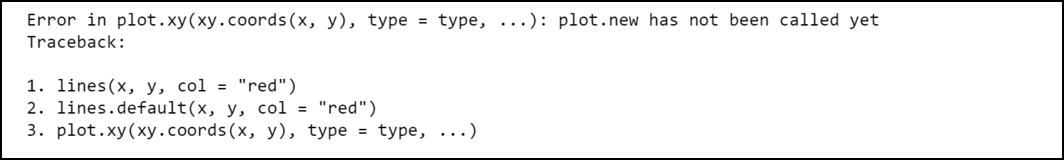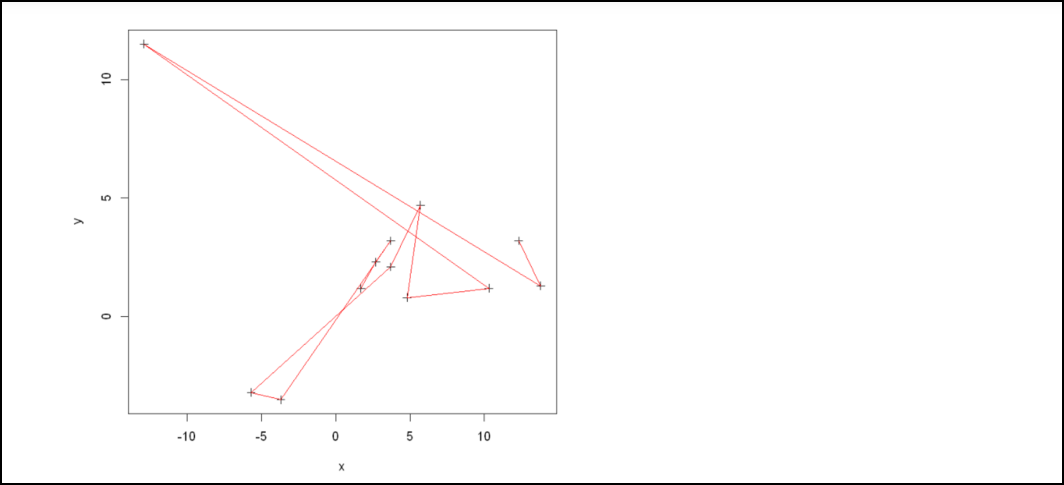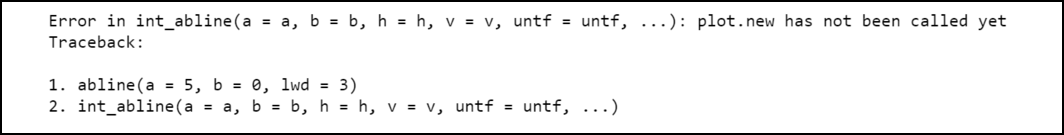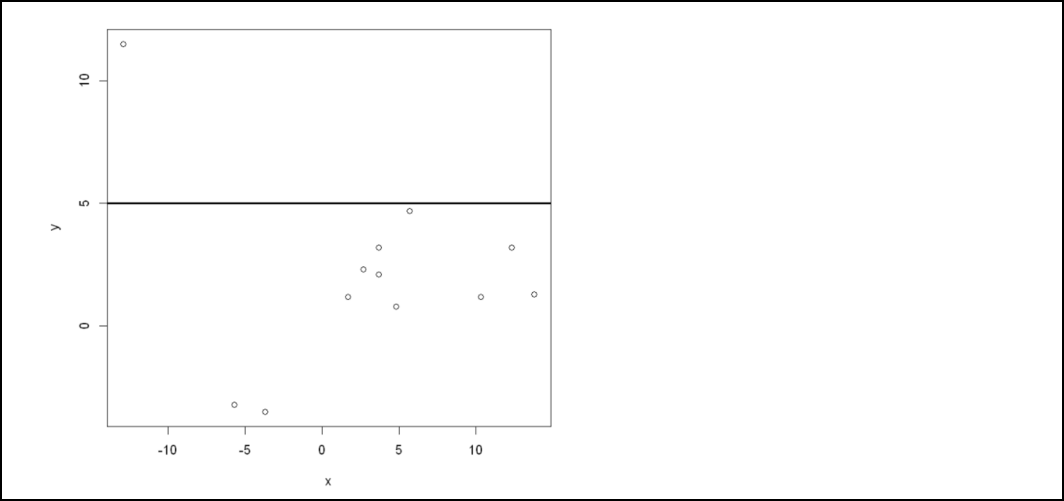GFG App
Open AppBrowser
Continue

# How to Fix in R: plot.new has not been called yet

In this article, we will discuss how to fix the “plot.new has not been called yet” error in the R programming language.

The R compiler produces such an error when we try to carry an operation that requires a plot to exist in R but the plot doesn’t exist yet.

```Error in plot.xy(xy.coords(x, y), type = type, ...) :
plot.new has not been called yet```

## Method 1: How to fix with lines() function

Here we will focus on how we can fix the error that can be produced by the R compiler while dealing with the lines() function.

Example:

Let’s consider an example, we have two vectors that hold the corresponding X and Y coordinates of 12 different points. Then we have used the lines() function to draw lines joining the points.

## R

 `# R program to add lines into plots` ` `  `# Constructing coordinate vectors` `x <- ``c``(1.7, 2.7, 3.7, -3.7, -5.7,` `       ``3.7, 5.7, 4.8, 10.3, -12.9, 13.8, 12.3)` `y <- ``c``(1.2, 2.3, 3.2, -3.5, -3.2, 2.1,` `       ``4.7, .8, 1.2, 11.5, 1.3, 3.2)`   `# Trying to draw lines of red colors` `lines``(x, y, col = ``"red"``)`

Output:The R compiler produces such an error because we didn’t create the plot before using the lines() function. We can fix this error easily by creating a plot before using the lines() function:

Example:

Here, in this example, we are fixing the above error by simply calling the plot function before the lines() function to get the idea of the points given.

## R

 `# R program to add lines into plots` ` `  `# Constructing coordinate vectors` `x <- ``c``(1.7, 2.7, 3.7, -3.7, -5.7,` `       ``3.7, 5.7, 4.8, 10.3, -12.9, 13.8, 12.3)` `y <- ``c``(1.2, 2.3, 3.2, -3.5, -3.2, 2.1,` `       ``4.7, .8, 1.2, 11.5, 1.3, 3.2)` ` `  `# Plotting the graph` `plot``(x, y, cex = 1, pch = 3,` `     ``xlab =``"x"``, ylab =``"y"``,` `     ``col =``"black"``)`   `# Trying to draw lines of red colors` `lines``(x, y, col = ``"red"``)`

Output:This time the program compiled successfully because we have created the plot before using the lines() function.

## Method 2: How to fix with abline() function:

In this part, we focus on how we can fix the error that can be produced by the R compiler while dealing with the abline() function.

Example:

Let’s consider an example, we have two vectors that hold the corresponding X and Y coordinates of 12 different points. Then we have used the ablines() function to draw a horizontal line at Y = 5.

## R

 `# R program to add a horizontal line` ` `  `# Constructing coordinate vectors` `x <- ``c``(1.7, 2.7, 3.7, -3.7, -5.7,` `       ``3.7, 5.7, 4.8, 10.3, -12.9, 13.8, 12.3)` `y <- ``c``(1.2, 2.3, 3.2, -3.5, -3.2, 2.1,` `       ``4.7, .8, 1.2, 11.5, 1.3, 3.2)`   `# Try to add horizontal line at y=5` `abline``(a=5, b=0, lwd=3)`

Output:The R compiler produces such an error because we didn’t create the plot before using the abline() function. We can fix this error easily by creating a plot before using the abline() function:

Example:

So, here for resolving the above error, we are calling the plot() function before the abline function with the given parameters, and by this, the program compiled successfully without any error.

## R

 `# R program to add a horizontal line` ` `  `# Constructing coordinate vectors` `x <- ``c``(1.7, 2.7, 3.7, -3.7, -5.7,` `       ``3.7, 5.7, 4.8, 10.3, -12.9, 13.8, 12.3)` `y <- ``c``(1.2, 2.3, 3.2, -3.5, -3.2, 2.1,` `       ``4.7, .8, 1.2, 11.5, 1.3, 3.2)` ` `  `# Plotting the graph` `plot``(x, y, cex = 1, pch = 3,` `     ``xlab =``"x"``, ylab =``"y"``,` `     ``col =``"black"``)`   `#attempt to add horizontal line at y=5` `abline``(a=5, b=0, lwd=3)`

Output:My Personal Notes arrow_drop_up